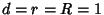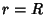## LensA figure composed of two equal and symmetrically placed circular Arcs. It is also known as the Fish Bladder (Pedoe 1995, p. xii) or Vesica Piscis. The latter term is often used for the particular lens formed by the intersection of two unit Circles whose centers are offset by a unit distance (Rawles 1997). In this case, the height of the lens is given by lettingin the equation for a Circle-Circle Intersection(1)

giving. The Area of the Vesica Piscis is given by plugginginto the Circle-Circle Intersection area equation with,(2)

giving(3)

Renaissance artists frequently surrounded images of Jesus with the vesica piscis (Rawles 1997). An asymmetrical lens is produced by a Circle-Circle Intersection for unequal Circles.

See also Circle, Circle-Circle Intersection, Flower of Life, Lemon, Lune (Plane), Reuleaux Triangle, Sector, Seed of Life, Segment, Venn Diagram

References

Pedoe, D. Circles: A Mathematical View, rev. ed. Washington, DC: Math. Assoc. Amer., 1995.

Rawles, B. Sacred Geometry Design Sourcebook: Universal Dimensional Patterns. Nevada City, CA: Elysian Pub., p. 11, 1997.